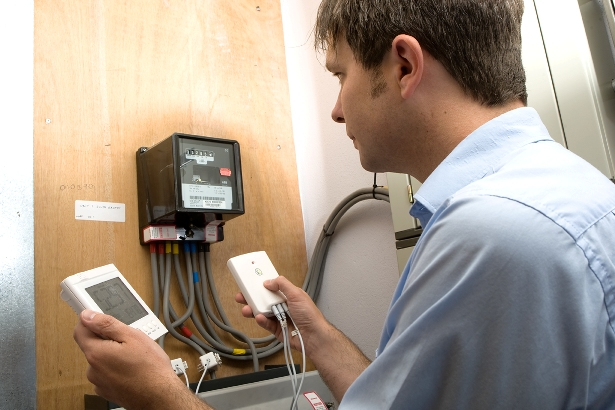# Fundamentals of Power Factor CalculatorThe power factor calculation is mostly for the electrical engineers who have knowledge about electricity. But the calculations can be performed by common people who know some of the fundamentals of electricity. Most of the understandings of some individuals stop at a point that the electricity turns the equipments into one state. But that is the end result and there is much more besides this. The most important aspect about electricity is that it is constant and pure in itself.

Fundamental concepts of power factor:
Before moving to the calculation of power factor one should know the fundamentals of power factor like what is it? Power factor for AC is defined as the real power flowing towards the load to that of the apparent power within the circuit. It is mostly a dimensionless number that ranges between -1 and +1. Real power is mostly the capacity of the circuit that performs work in a particular interval of time whereas apparent power is the product of voltage and current in the circuit. The apparent power will be more than the real power due to the energy stored within the load and also returned to a source. It may be due to the non linear load that somehow distorts the shape of the wave of the drawn current from the source. In an electrical power system, a load having low power factor draws maximum current than the load with higher power factor for the same amount of transferred useful power.

The power triangle is the ratio between the amounts of power consumed to that the amount of power absorbed. It is the same angle as that of the circuit’s impedance in its polar form. The true power and the apparent power form the adjacent and hypotenuse sides of one right angled triangle. The ratio of power factor is equal to the cosine of the phase angle. For a pure resistive circuit the power factor remains 1 as the reactive power is 0. Using values:

Power factor= true power/ apparent power
Power factor= 119.365w/169.256va
Power factor= 0.705Facility power is the dirty electricity which surges and fluctuates. Surging power is bad for the sensitive electronic equipments and also can ruin those equipments. Electronics are not cheap things and can’t be replaced so easily. We have solved the problem, but the question arises how? Answer is quite simple. This problem can be resolved by power calculation. And for the calculation we require a power factor calculator.

This helps to assess how much the equipments are pulling the power and how much they can pull. This helps to know the maximum extent of the power and also to make sure about the safe distribution. There are units that can make a perfect sine wave as output and can clean the facility power. This means there will be no fluctuation which causes electronics to last longer. This helps to save of electronic equipments that are expensive and can’t be easily replaced. The best way is to use the electric saver power 1200unit. This saves energy and also helps in reduction of the electric bill for the common people. It has proven to be far more beneficial than any other equipment.

Want to Start Saving Money and Power the Easy Way?  Click The Buy Now Button below:Having a proper power factor calculation may help to resolve many problems. So its better to stop dealing with the hazardous facility electricity and take proper steps that are necessary for personal assets.0
Shares